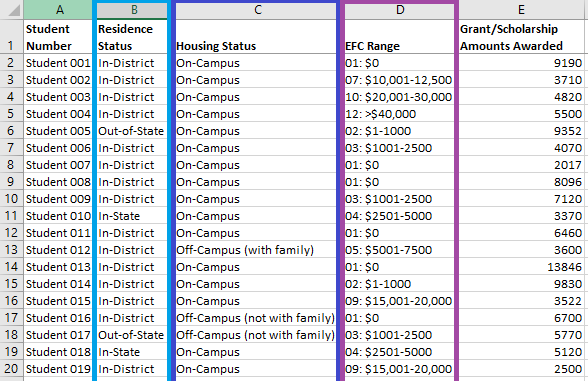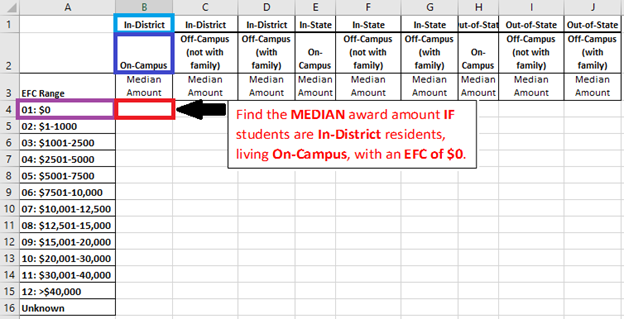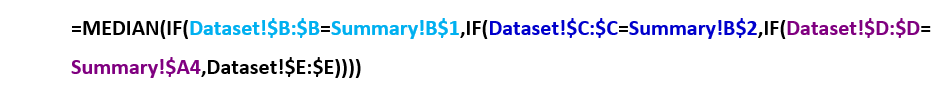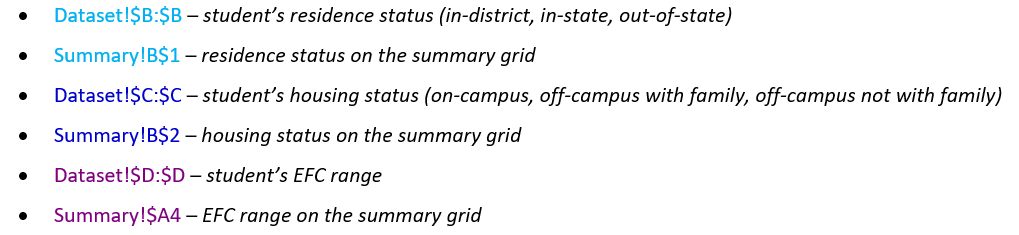# Tips & Insights

Tips & Insights provides advice on and examples of technology used to simplify and streamline IR activities, as well as real world examples of visual displays of data (e.g., charts, graphs, maps, tables, pictures). These eAIR features are developed by members.

• Tech Tips
• 10.15.19

# Excel MEDIAN IF Array Formula

A MEDIAN IF array formula in Excel will identify the middle number of values that meet certain criteria. An array formula performs an operation on multiple values instead of a single value. In this array formula, we essentially filter the dataset using the formula so that we find the median of only the values that meet our conditions. This formula can be used to complete the College Board’s Net Price Calculator.

### Basics

The syntax and arguments for the MEDIAN IF formula are as follows:

{=MEDIAN(IF(logical_test,value_if_true,value_if_false)}

Purposes of each part of the formula:

• The MEDIAN function finds the middle value out of a set of numbers.
• The IF function allows us to set conditions for the values we want to examine.
• The array formula lets the IF function test for multiple conditions in a single cell. When the condition is met, the array formula determines what data the MEDIAN function will examine to find the middle award amount.

To complete an array formula, type the formula (minus the curly brackets), then press Control, Shift, and Enter at the same time. This will finalize the array formula in Excel and insert the curly brackets at the beginning and end of the formula. Do not type the curly brackets in the formula. Array formulas that are created by entering Control, Shift, and Enter are also known as CSE formulas.

### Example

The MEDIAN IF formula is useful for calculating the median grant/scholarship amounts awarded to students fitting various residency, housing, and estimated family contribution (EFC) criteria that are required to complete the Net Price Calculator template that estimates net costs for students.

### Method

In this example, we be working with two worksheets in our Excel workbook:

1. Dataset – this worksheet includes the fictitious data we will use to calculate our median award amounts (see Figure 1). It includes the student ID, residence status, housing status, EFC range, and grant/scholarship amounts awarded. Note that Figure 1 shows only a portion of the dataset to illustrate what data elements are included.
2. Summary – this worksheet includes a summary grid in which to pull information from the Dataset worksheet (see Figure 2)

Figure 1. Dataset Worksheet (only a portion of the dataset is shown)Figure 2. Summary Worksheet - Net Price Calculator GridTo begin, use a MEDIAN IF array formula to find the median award amount for students who reside in-district, live on-campus, and have an EFC of \$0 (see red box in Figure 2, which is cell B4). The light blue, dark blue, and purple boxes are the criteria for the formula.

In cell B4 of the Summary worksheet, enter the following formula:The following column and cell references represent our criteria:For the students for which the above criteria are met, the median is taken of the following column:

• Dataset!\$E:\$E – student’s grant/scholarship amount awarded

The \$ in the location reference freezes the specified column or row reference, so that when the formula is copied and pasted into new cells, the frozen references remain the same.

To complete the array formula, press Control, Shift, and Enter at the same time (curly brackets will be automatically inserted to enclose the formula).

To populate the rest of the summary grid, copy and paste the formula into all cells of the grid.

In narrative form, this formula says, “Return the median of grant/scholarship award amount values for students whose residence status matches the residence status selected on the summary grid AND whose housing status matches the housing status selected on the summary grid AND whose EFC range matches the EFC range selected on the summary grid.”

### General Array Formula Tips

Array formulas can take longer to calculate and increase file sizes more than non-array formulas. To address these issues, consider doing the following:

• Copy and paste array formulas into new cells in small sections at a time (for example, if you need to copy an array formula into a total of 800 cells, split your process up so that you copy the formula into 200 cells at a time).
• After pasting the array formula into cells, copy the results and paste special: values into the same location. This will remove the formulas, so that they do not continue adding to the file size and processing speed.

A similar process using array formulas can be used to find the maximum or minimum of values that meet conditions you specify.

• {=MAX(IF(logical_test,value_if_true,value_if_false)}
• {=MIN(IF(logical_test,value_if_true,value_if_false)}
Back to Tips & Insights